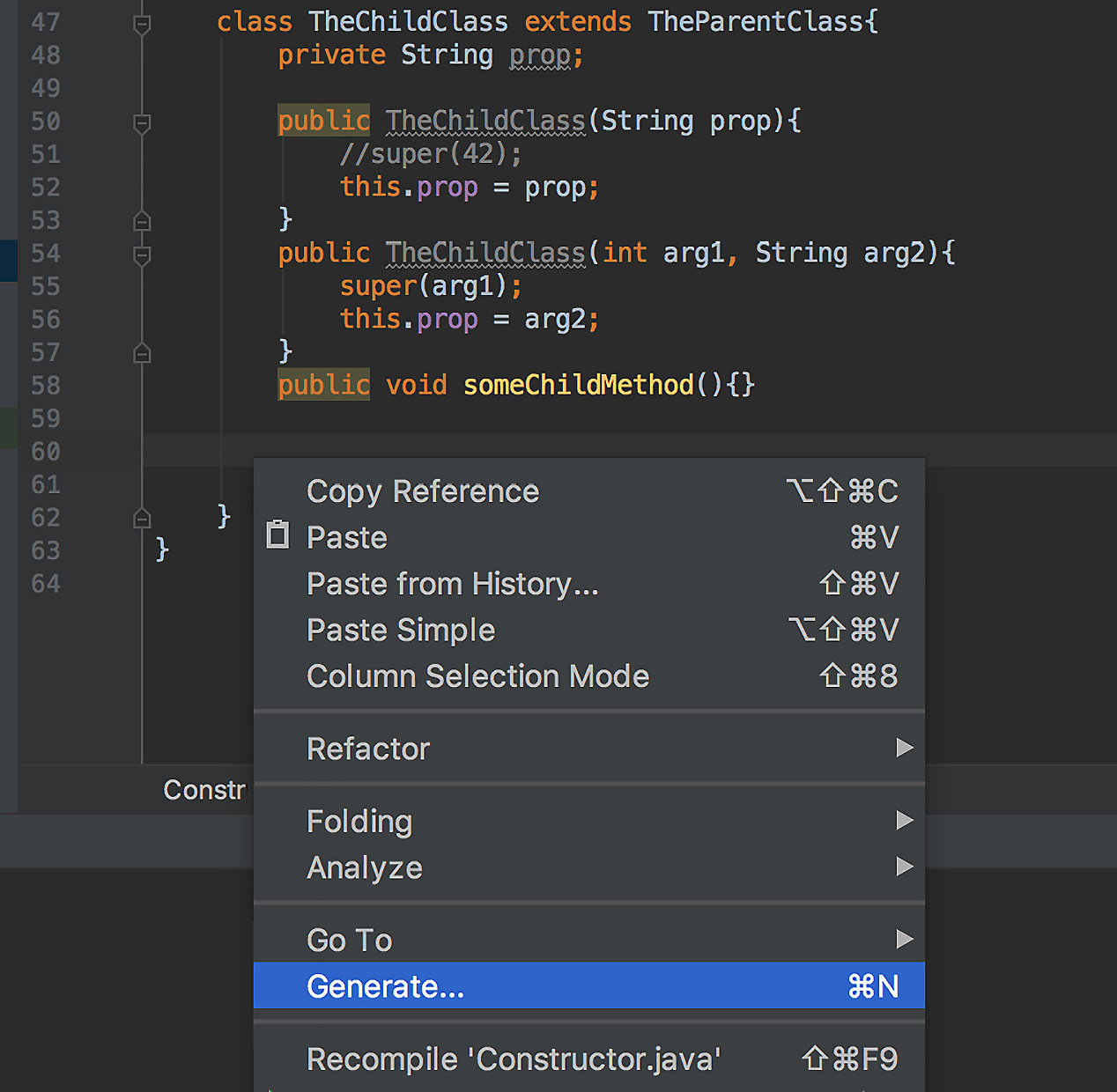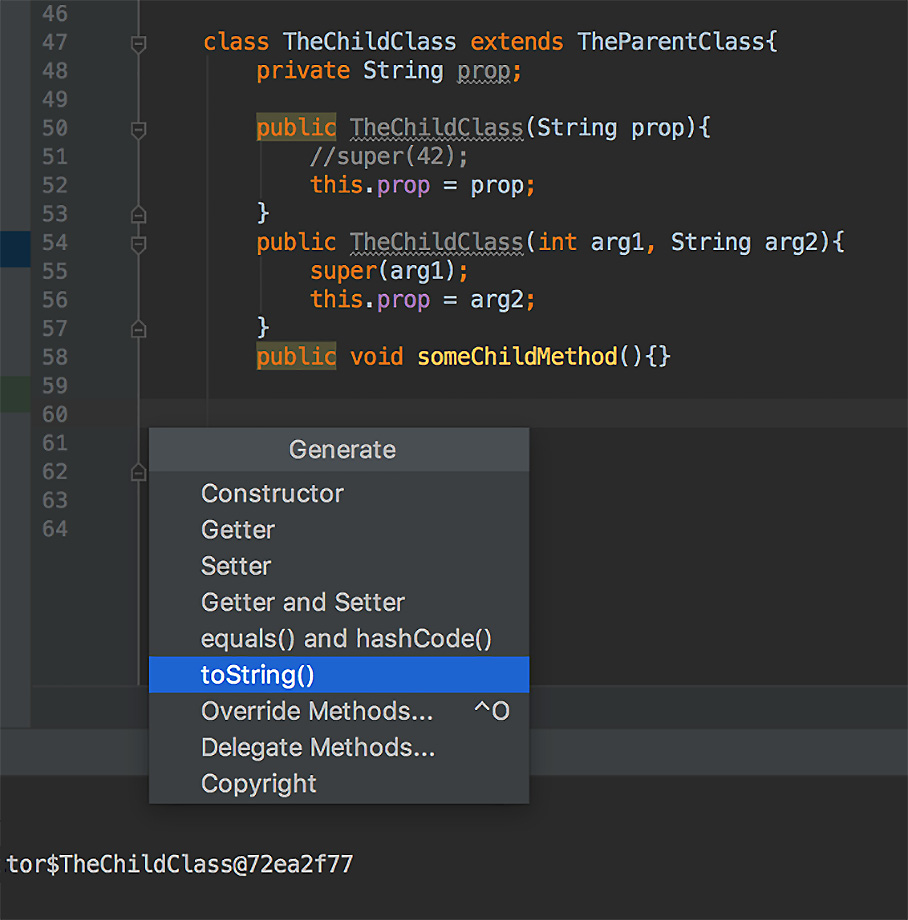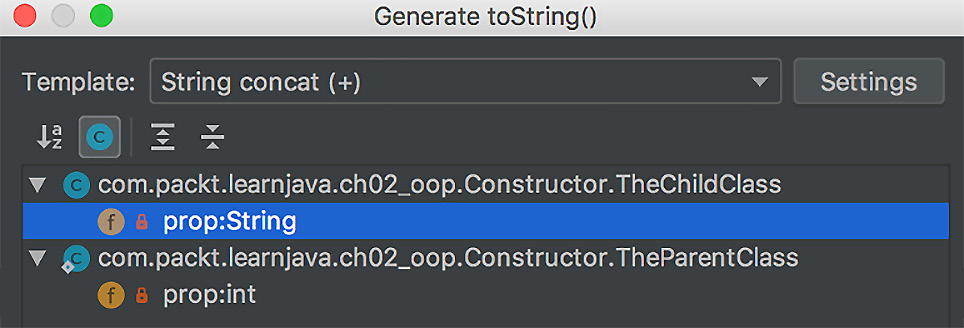# Chapter 2: Java Object-Oriented Programming (OOP)

• 面向对象的概念
• 班级
• 界面
• 重载、覆盖和隐藏
• final 变量、方法和类
• 记录和密封类
• 多态性在行动

# Technical requirements

• 装有 Microsoft Windows、Apple macOS 或 Linux 操作系统的计算机
• Java SE 版本 17 或更高版本
• 您喜欢的 IDE 或代码编辑器

# OOP concepts

• 类：定义对象的属性和行为（方法）是基于这个类创建的。
• 对象：这将状态（数据）定义为其属性的值，添加所采取的行为（方法） 来自一个类，并将它们保持在一起。
• 继承：这会将行为沿通过父级连接的类的链传播孩子的关系。
• 接口：这描述了如何 访问对象数据和行为。它将对象的外观 与其实现（行为）隔离（抽象）。
• 封装：隐藏状态实现的细节。
• 多态性：这允许对象呈现 已实现 接口的外观和表现得像任何祖先类。

## Inheritance

Java 中的父子关系使用 `extends` 关键字表示：

``class A { }``
``class B extends A { }``
``class C extends B { }``
``class D extends C { }``

• `D` 类继承自 `A``B``C` 类。
• `C` 类继承自 `A``B` 类。
• `B` 类继承自 `A` 类。

`A` 类的所有非私有方法都由 `B``C``D` 类。

`B` 类的所有非私有方法都由 `C``D` 类。

`C` 类的所有非私有方法都由 `D` 类继承（如果没有被覆盖）。

## Abstraction/interface

``interface A { }``
``interface B extends A {}``
``interface C extends B {}``
``interface D extends C {}``

• `D` 接口继承自 `A``B``C` 接口。
• `C` 接口继承自 `A``B` 接口。
• `B` 接口继承自 `A` 接口。

`A`接口的所有非私有方法都被`B````C 和 D 接口。```

`B` 接口的所有非私有方法都被 `C``D` 继承代码>接口。

`C` 接口的所有非私有方法都由 `D` 接口继承。

## Encapsulation

``class A {``
``  private String prop = "init value";``
``  public void setProp(String value){``
``     prop = value;``
``  }``
``  public String getProp(){``
``     return prop;``
``  }``
``}``

# Class

Java 程序 是表示可执行操作的语句序列。语句按方法组织，方法按类组织。一个或多个类存储在 `.java` 文件中。它们可以由 `javac` Java 编译器编译（从 Java 语言转换为字节码）并存储在 `.class` 文件中。每个`.class`文件只包含一个编译好的类，可以被JVM执行。

`java` 命令启动 JVM 并告诉它哪个类是 `main` 类，具有称为 `main()``main` 方法有一个特定的声明——它必须是 `public static`，必须返回 `void `，名称为 `main`，并接受 `String` 类型数组的单个参数。

JVM 将主类加载到内存中，找到 `main()` 方法，并开始逐句执行它。 `java` 命令还可以传递 `main()` 方法作为 `字符串`值。如果 JVM 遇到需要执行另一个类的方法的语句，该类（它的 `.class` 文件）也会被加载到内存中并执行相应的方法。因此，Java 程序流程就是加载类并执行它们的方法。

``public class MyApp {``
``  public static void main(String[] args){``
``     AnotherClass an = new AnotherClass();``
``     for(String s: args){``
``        an.display(s);``
``     }``
``   }``
``}``

``public class AnotherClass {``
``   private int result;``
``   public void display(String s){``
``      System.out.println(s);``
``   }``
``   public int process(int i){``
``      result = i *2;``
``      return result;``
``   }``
``   public int getResult(){``
``      return result;``
``   }``
``} ``

• `process()` 方法将输入整数加倍，将其存储在其 `result` 属性中，并将值返回给调用者。
• `getResult()` 方法允许您在以后的任何时间从对象中获取结果。

`private` 关键字使值只能从类内部，从其方法访问。 `public` 关键字使任何其他类都可以访问属性或方法。

## Method

``<return type> <method name>(<list of parameter types>){``
``     <method body that is a sequence of statements>``
``}``

``double doSomething(String s, int i){``
``    //some code goes here``
``}``
``double doSomething(String i, int s){``
``    //some code other code goes here``
``}``

``double doSomething(String s, int i){``
``    //some code goes here``
``}``
``double doSomething(int s, String i){``
``    //some code other code goes here``
``}``

### Varargs

``String someMethod(String s, int i, double... arr){``
`` //statements that compose method body``
``}``

``public static void main(String... args){``
``    someMethod("str", 42, 10, 17.23, 4);``
``}``
``private static String someMethod(``
``        String s, int i, double... arr){``
``    System.out.println(arr + ", " + arr + ", " + arr); ``
``                                     //prints: 10.0, 17.23, 4.0``
``    return s;``
``}``

## Constructor

``class SomeClass {``
``     private int prop1;``
``     private String prop2;``
``     public SomeClass(int prop1){``
``         this.prop1 = prop1;``
``     }``
``     public SomeClass(String prop2){``
``         this.prop2 = prop2;``
``     }``
``     public SomeClass(int prop1, String prop2){``
``         this.prop1 = prop1;``
``         this.prop2 = prop2;``
``     }   ``
``     // methods follow ``
``}``

``class TheParentClass {``
``    private int prop;``
``    public TheParentClass(int prop){``
``        this.prop = prop;``
``    }``
``    // methods follow``
``}``
``class TheChildClass extends TheParentClass{``
``    private int x;``
``    private String prop;``
``    private String anotherProp = "abc";``
``    public TheChildClass(String prop){``
``       super(42);``
``       this.prop = prop;``
``    }``
``    public TheChildClass(int arg1, String arg2){``
``       super(arg1);``
``       this.prop = arg2;``
``    }``
``    // methods follow``
``}``

• 当对象及其任何父对象都没有需要初始化的属性时
• 当每个属性与类型声明一起初始化时（例如，`int x = 42`
• 当属性初始化的默认值足够好时

``class TheParentClass {``
``    private int prop;``
``    public TheParentClass(int prop){``
``        this.prop = prop;``
``    }``
``    // methods follow``
``}``
``class TheChildClass extends TheParentClass{``
``    private String prop;``
``    public TheChildClass(String prop){``
``        //super(42);  //No call to the parent's constructor``
``        this.prop = prop;``
``    }``
``    // methods follow``
``}``

``class TheParentClass {``
``    private int prop;``
``    public TheParentClass() {}``
``    public TheParentClass(int prop){``
``        this.prop = prop;``
``    }``
``    // methods follow``
``}``
``class TheChildClass extends TheParentClass{``
``    private String prop;``
``    public TheChildClass(String prop){``
``        this.prop = prop;``
``    }``
``    // methods follow``
``}``

## The new operator

`new` 操作符创建一个类的对象（也可以说它实例化一个类或创建一个类的实例），通过为新对象的属性分配内存并返回对该记忆的引用。此内存引用分配给与用于创建对象的类或其父对象的类型相同类型的变量：

``TheChildClass ref1 = new TheChildClass("something"); ``
``TheParentClass ref2 = new TheChildClass("something");``

``class TheParentClass {``
``    private int prop;``
``    public TheParentClass(int prop){``
``        this.prop = prop;``
``    }``
``    public void someParentMethod(){}``
``}``
``class TheChildClass extends TheParentClass{``
``    private String prop;``
``    public TheChildClass(int arg1, String arg2){``
``        super(arg1);``
``        this.prop = arg2;``
``    }``
``    public void someChildMethod(){}``
``}``

``TheChildClass ref1 = new TheChildClass("something");``
``TheParentClass ref2 = new TheChildClass("something");``
``ref1.someChildMethod();``
``ref1.someParentMethod();``
``((TheChildClass) ref2).someChildMethod();``
``ref2.someParentMethod();``

``TheParentClass ref2 = new TheParentClass(42);``
``((TheChildClass) ref2).someChildMethod();  //compiler's error``
``ref2.someParentMethod();``

``void someMethod(){``
``   SomeClass ref = new SomeClass();``
``   ref.someClassMethod();``
``   //other statements follow``
``}``

`someMethod()` 方法执行完成后，`SomeClass` 的对象不可访问 了。这就是垃圾收集器注意到的， 它释放了这个对象占用的内存。我们将在 Chapter 9 JVM 结构和垃圾收集

## Class java.lang.Object

``class A { }``
``class B extends A {}``
``class C extends B {}``
``class D extends C {}``

• `public String toString()`
• `public int hashCode()`
• `public boolean equals (Object obj)`
• `public Class getClass()`
• `受保护对象 clone()`
• `public void notify()`
• `public void notifyAll()`
• `public void wait()`
• `public void wait(long timeout)`
• `public void wait(long timeout, int nanos)`

``public String toString() {``
``   return getClass().getName()+"@"+``
``                     Integer.toHexString(hashCode());``
``}``

``TheChildClass ref1 = new TheChildClass("something");``
``System.out.println(ref1.toString());  ``
``//prints: com.packt.learnjava.ch02_oop.``
``//[email protected]````@Override``
``public String toString() {``
``    return "TheChildClass{" +``
``            "prop='" + prop + '\'' +``
``            '}';``
``}``

``TheChildClass ref1 = new TheChildClass("something");``
``System.out.println(ref1.toString());  ``
``                      //prints: TheChildClass{prop='something'}``

`hashCode()``equals()` 方法将在 第 6 章数据结构，泛型和流行的实用程序

`getClass()``clone()` 方法不常用。 `getClass()` 方法返回 `Class` 类的对象，该对象具有许多提供各种系统信息的方法。最常用的方法是返回当前对象的类名的方法。 `clone()` 方法可用于复制当前的 对象。只要当前对象 的所有属性都是原始类型，它就可以正常工作。但是，如果存在引用类型属性，则必须重新实现 `clone()` 方法，以便正确完成引用类型的复制。否则，只会复制引用，而不是对象本身。这样的副本称为浅副本，在某些情况下可能已经足够了。 `protected` 关键字表示只有类的子级可以访问它。请参阅包、导入和访问部分。

`Object` 类的最后五个方法用于线程之间的通信——用于并发处理的轻量级进程。它们通常不会重新实现。

## Instance and static properties and methods

``class SomeClass{``
``    public static void someMethod(int i){``
``        //do something``
``    }``
``}``

``SomeClass.someMethod(42);``

``class SomeClass{``
``    public static String SOME_PROPERTY = "abc";``
``}``

``System.out.println(SomeClass.SOME_PROPERTY);  //prints: abc``

• 存储一个常量——一个可以读取但不能修改的值（也称为只读值）
• 存储创建成本高或保留只读值的无状态对象

``class SomeClass{``
``    public static final String INPUT_FILE_NAME = "myFile.csv";``
``}``

``class SomeClass{``
``    public static String someMethod() {``
``        return "abc";``
``    }``
``}``

``System.out.println(SomeClass.someMethod()); //prints: abc``

# Interface

Abstraction/interface 部分，我们用一般的 术语讨论了接口。在本节中，我们将描述一种表达它的 Java 语言结构。

``interface SomeInterface {``
``    void method1();``
``    String method2(int i);``
``}``

``class SomeClass implements SomeInterface{``
``    public void method1(){``
``        //method body``
``    }``
``    public String method2(int i) {``
``        //method body``
``        return "abc";``
``    }``
``}``

``SomeInterface si = new SomeClass(); ``

## Default methods

``interface SomeInterface {``
``    void method1();``
``    String method2(int i);``
``    default int method3(){``
``        return 42;``
``    }``
``}``
``class SomeClass implements SomeInterface{``
``    public void method1(){``
``        //method body``
``    }``
``    public String method2(int i) {``
``        //method body``
``        return "abc";``
``    }``
``}``

``SomeClass sc = new SomeClass();``
``sc.method1();``
``sc.method2(22);  //returns: "abc"``
``System.out.println(sc.method2(22)); //prints: abc``
``sc.method3();    //returns: 42``
``System.out.println(sc.method3());   //prints: 42``

``class SomeClass implements SomeInterface{``
``    public void method1(){``
``        //method body``
``    }``
``    public String method2(int i) {``
``        //method body``
``        return "abc";``
``    }``
``    public int method3(){``
``        return 15;``
``    }``
``}``

``SomeClass sc = new SomeClass();``
``sc.method1();``
``sc.method2(22);  //returns: "abc"``
``sc.method3();    //returns: 15``
``System.out.println(sc.method3());      //prints: 15``

## Private methods

``interface SomeInterface {``
``    void method1();``
``    String method2(int i);``
``    default int method3(){``
``        return getNumber();``
``    }``
``    default int method4(){``
``        return getNumber() + 22;``
``    }``
``    private int getNumber(){``
``        return 42;``
``    }``
``}``

## Static fields and methods

``interface SomeInterface{``
``   static String someMethod() {``
``      return "abc";``
``   }``
``}``

``System.out.println(SomeInetrface.someMethod()); //prints: abc``

## Interface versus abstract class

• abstract 类可以有构造函数，而接口 不能。
• 抽象类可以有状态，而接口不能。
• 抽象类的字段可以是 `public``private``protected` , `static` 与否，以及 `final` 与否，而在接口中，字段始终是 `public``static``final`
• 抽象类中的方法可以是 `public``private``protected` ，而接口方法只能是 `public``private`
• 如果您要修改的类已经扩展了另一个类，则不能使用抽象类，但可以实现接口，因为一个类只能扩展另一个类，但可以实现多个接口。

``interface A {``
``    int m(String s);``
``    int m(String s, double d);``
``    default int m(String s, int i) { return 1; }``
``    static int m(String s, int i, double d) { return 1; }``
``}``

``    class C {``
``        int m(String s){ return 42; }``
``        int m(String s, double d){ return 42; }``
``        static int m(String s, double d, int i) { return 1; }``
``    }``

``interface A {``
``    int m(String s);``
``    int m(String s, double d);``
``}``
``interface B extends A {``
``    default int m(String s, int i) { return 1; }``
``    static int m(String s, int i, double d) { return 1; }``
``}``
``class C {``
``     int m(String s){ return 42; }``
``}``
``class D extends C {``
``     int m(String s, double d){ return 42; }``
``     static int m(String s, double d, int i) { return 1; }``
``}``

## Overriding

``interface A {``
``    default void method(){``
``        System.out.println("interface A");``
``    }``
``}``
``interface B extends A{``
``    @Override``
``    default void method(){``
``        System.out.println("interface B");``
``    }``
``}``
``class C implements B { }``

``C c = new C();``
``c.method();      //prints: interface B``

``interface B extends A{``
``    @Override``
``    default void method(){``
``        System.out.println("interface B");``
``    }``
``}``

``C c = new C();``
``c.method();      //prints: interface A``

``class C1{``
``    public void method(){``
``        System.out.println("class C1");``
``    }``
``}``
``class C2 extends C1{``
``    @Override``
``    public void method(){``
``        System.out.println("class C2");``
``    }``
``}``

``C2 c2 = new C2();``
``c2.method();      //prints: class C2``

``class C1{``
``    public void method(){``
``        System.out.println("class C1");``
``    }``
``}``
``class C3 extends C1{``
``    public void someOtherMethod(){``
``        System.out.println("class C3");``
``    }``
``}``
``class C2 extends C3{``
``    @Override``
``    public void method(){``
``        System.out.println("class C2");``
``    }``
``}``

## Hiding

hiding 这个名字来源于类和接口的静态属性和方法的行为。每个静态属性或方法在 JVM 内存中作为单个副本存在，因为它们与接口或类相关联，而不是与对象相关联。接口或类作为单个副本存在。这就是为什么我们不能说子类的静态属性或方法会覆盖父类的同名静态属性或方法。所有静态属性 和方法仅在加载类或接口时加载到内存中并保留在那里，不会被复制到任何地方。让我们看一个例子。

``interface A {``
``    String NAME = "interface A";``
``    static void method() {``
``        System.out.println("interface A");``
``    }``
``}``
``interface B extends A {``
``    String NAME = "interface B";``
``    static void method() {``
``        System.out.println("interface B");``
``    }``
``}``

``System.out.println(B.NAME); //prints: interface B``
``B.method();                 //prints: interface B``

``public class C {``
``    public static String NAME = "class C";``
``    public static void method(){``
``        System.out.println("class C"); ``
``    }``
``    public String name1 = "class C";``
``}``
``public class D extends C {``
``    public static String NAME = "class D";``
``    public static void method(){``
``        System.out.println("class D"); ``
``    }``
``    public String name1 = "class D";``
``}``

``System.out.println(D.NAME);  //prints: class D``
``D.method();                  //prints: class D``

``C obj = new D();``
``System.out.println(obj.NAME);        //prints: class C``
``System.out.println(((D) obj).NAME);  //prints: class D``
``obj.method();                        //prints: class C``
``((D)obj).method();                   //prints: class D``
``System.out.println(obj.name1);       //prints: class C``
``System.out.println(((D) obj).name1); //prints: class D``

`obj` 变量引用 `D` 类的对象，铸造证明了这一点，正如您在前面的示例中看到的那样。但是，即使我们使用对象，尝试访问静态属性或方法也会为我们带来 我们用作声明变量类型的类的成员。至于例子最后两行的实例属性，Java中的属性不符合多态行为，我们得到父`name1`属性"literal">C 类，而不是子 `D` 类的预期属性。

``class X {``
``    private String name = "class X";``
``    public String getName() {``
``        return name;``
``    }``
``    public void setName(String name) {``
``        this.name = name;``
``    }``
``}``
``class Y extends X {``
``    private String name = "class Y";``
``    public String getName() {``
``        return name;``
``    }``
``    public void setName(String name) {``
``        this.name = name;``
``    }``
``}``

``X x = new Y();``
``System.out.println(x.getName());      //prints: class Y``
``System.out.println(((Y)x).getName()); //prints: class Y``

``public class HidingProperty {``
``   private static String name1 = "static property";``
``   private String name2 = "instance property";``
``   public void method() {``
``      var name1 = "local variable";``
``      System.out.println(name1);     //prints: local variable``
``      var name2 = "local variable";  //prints: local variable``
``      System.out.println(name2);``
``      System.out.println(HidingProperty.name1); ``
``                                     //prints: static property``
``      System.out.println(this.name2);``
``                                   //prints: instance property``
``   }``
``}``

# The final variable, method, and classes

## The final variable

`final` 关键字放在变量声明前面 使这个变量在初始化后不可变，例如：

``final String s = "abc";``

``final String s;``
``s = "abc";``

``class A {``
``    private final String s1 = "abc";``
``    private final String s2;``
``    private final String s3;   //error``
``    private final int x;       //error``
``    public A() {``
``        this.s1 = "xyz";      //error``
``        this.s2 = "xyz";     ``
``    }``
``}``

``class B {``
``    private final String s1 = "abc";``
``    private final String s2;``
``    {``
``        s1 = "xyz"; //error``
``        s2 = "abc";``
``    }``
``}``

``class C {``
``    private final static String s1 = "abc";``
``    private final static String s2;``
``    static {``
``        s1 = "xyz"; //error``
``        s2 = "abc";``
``    }``
``}``

``interface I {``
``    String s1;  //error``
``    String s2 = "abc";``
``}``

## Final method

``public final Class getClass()x``
``public final void notify()``
``public final void notifyAll()``
``public final void wait() throws InterruptedException``
``public final void wait(long timeout) ``
``                         throws InterruptedException``
``public final void wait(long timeout, int nanos)``
``                         throws InterruptedException``

`final` 类的所有私有方法和未继承方法实际上都是最终方法，因为您无法覆盖它们。

## Final class

`final` 类不能扩展。它不能有孩子，这使得类的所有方法 也有效地`final`。此功能用于安全性或当程序员希望确保类功能不会因为其他一些设计考虑而被覆盖、重载或隐藏时。

# The record class

`record` 在 Java 16 中添加到 SDK。这是一个期待已久的 Java 功能。它允许您在需要不可变类（仅使用 getter）时避免编写样板代码，该类类似于以下 `Person` 类（参见 `ch02_oop` 文件夹中的 "literal">Record 类）：

``final class Person {``
``    private int age;``
``    private String name;``
``    public Person(int age, String name) {``
``        this.age = age;``
``        this.name = name;``
``    }``
``    public int age() { return age; }``
``    public String name() { return name; }``
``    @Override``
``    public boolean equals(Object o) {``
``        //implementation not shown for brevity``
``    }``
``    @Override``
``    public int hashCode() {``
``        //implementation not shown for brevity``
``    }``
``    @Override``
``    public String toString() {``
``        //implementation not shown for brevity``
``    }``

`record` 类只允许您将前面的实现替换为以下一行：

``record Person(int age, String name){}``

``record PersonR(int age, String name){} //We added suffix "R" ``
``                 //to distinguish this class from class Person``
``Person person = new Person(25, "Bill");``
``System.out.println(person);  ``
``                          //prints: Person{age=25, name='Bill'}``
``System.out.println(person.name());            //prints: Bill``
`` ``
``Person person1 = new Person(25, "Bill");``
``System.out.println(person.equals(person1));   //prints: true``
`` ``
``PersonR personR = new PersonR(25, "Bill");``
``System.out.println(personR);   ``
``                         //prints: PersonR{age=25, name='Bill'}``
``System.out.println(personR.name());           //prints: Bill``
`` ``
``PersonR personR1 = new PersonR(25, "Bill");``
``System.out.println(personR.equals(personR1)); //prints: true``
`` ``
``System.out.println(personR.equals(person));   //prints: false``

``interface Student{``
``    String getSchoolName();``
``}``
``record StudentImpl(String name, String school) implements Student{``
``    @Override``
``    public String getSchoolName() { return school(); }``
``}``

``record StudentImpl(String name, String school) implements Student{``
``    public static String getSchoolName(Student student) {``
``         return student.getSchoolName();``
``    }``
``}``

`static` 方法不会也不能访问实例属性，并且只能使用作为参数传递给它的值。

`record` 可以有另一个构造函数，可以添加，例如如下：

``record StudentImpl(String name, String school) implements Student{``
``    public StudentImpl(String name) {``
``        this(name, "Unknown");``
``    } ``
``}``

# Sealed classes and interfaces

`final` 类不能扩展，而非公共类或接口的访问权限有限。然而，有时 需要从任何地方访问类或接口 但只能由某个类或接口扩展，或者，在接口的情况下，仅由某些类实现。这就是在 Java 17 中将 `sealed` 类和接口添加到 SDK 的动机。

`sealed` 类或接口与 `final` 的区别在于 ```sealed 类或接口总是有一个 permits 关键字，后面是允许扩展 sealed 类或接口，或者，在接口的情况下，实现它。请注意现有这个词。 permits 关键字之后列出的子类型必须在编译时与密封类存在于同一模块中，如果在默认（未命名）模块中，则必须存在于同一包中。```

`sealed` 类的子类型必须标记为 `sealed``final`、或 `非密封``sealed` 接口的子类型必须标记为 `sealed``非密封`，因为接口不能是`final`

``sealed interface Engine permits EngineBrand {``
``    int getHorsePower();``
``}``
``sealed interface EngineBrand extends Engine permits Vehicle {``
``    String getBrand();``
``} ``
``non-sealed class Vehicle implements EngineBrand {``
``    private final String make, model, brand;``
``    private final int horsePower;``
``    public Vehicle(String make, String model, ``
``                   String brand, int horsePower) {``
``        this.make = make;``
``        this.model = model;``
``        this.brand = brand;``
``        this.horsePower = horsePower;``
``    }``
``    public String getMake() { return make; }``
``    public String getModel() { return model; }``
``    public String getBrand() { return brand; }``
``    public int getHorsePower() { return horsePower; }``
``}``

``sealed interface Engine permits EngineBrand, Vehicle {``
``    int getHorsePower();``
``}``
``sealed interface EngineBrand extends Engine permits Vehicle {``
``    String getBrand();``
``} ``
``non-sealed class Vehicle implements Engine, EngineBrand {...}``

``sealed class Vehicle permits Car, Truck {``
``    private final String make, model;``
``    private final int horsePower;``
``    public Vehicle(String make, String model, int horsePower) {``
``        this.make = make;``
``        this.model = model;``
``        this.horsePower = horsePower;``
``    }``
``    public String getMake() { return make; }``
``    public String getModel() { return model; }``
``    public int getHorsePower() { return horsePower; }``
``}``

``final class Car extends Vehicle {``
``    private final int passengerCount;``
``    public Car(String make, String model, int horsePower, ``
``      int passengerCount) {``
``        super(make, model, horsePower);``
``        this.passengerCount = passengerCount;``
``    }``
``    public int getPassengerCount() { return passengerCount; }``
``}``
`` ``
``non-sealed class Truck extends Vehicle {``
``    private final int payloadPounds;``
``    public Truck(String make, String model, int horsePower, ``
``      int payloadPounds) {``
``        super(make, model, horsePower);``
``        this.payloadPounds = payloadPounds;``
``    }``
``    public int getPayloadPounds() { return payloadPounds; }``
``}``

``Vehicle vehicle = new Vehicle("Ford", "Taurus", 300);``
``System.out.println(vehicle.getClass().isSealed());  ``
``                                                 //prints: true``
``System.out.println(Arrays.stream(vehicle.getClass()``
``                .getPermittedSubclasses())``
``                .map(Objects::toString).toList());``
``                             //prints list of permitted classes``
`` ``
``Car car = new Car("Ford", "Taurus", 300, 4);``
``System.out.println(car.getClass().isSealed());  //prints: false``
``System.out.println(car.getClass().getPermittedSubclasses());``
``                                                 //prints: null``

`sealed` 接口与 `record` 集成得很好，因为 `record``final` 并且可以被列为 作为允许的 实现。

# Polymorphism in action

## The object factory

``interface CalcSomething{ double calculate(); }``
``class CalcUsingAlg1 implements CalcSomething{``
``    public double calculate(){ return 42.1; }``
``}``
``class CalcUsingAlg2 implements CalcSomething{``
``    private int prop1;``
``    private double prop2;``
``    public CalcUsingAlg2(int prop1, double prop2) {``
``        this.prop1 = prop1;``
``        this.prop2 = prop2;``
``    }``
``    public double calculate(){ return prop1 * prop2; }``
``}``

``class CalcFactory{``
``    public static CalcSomething getCalculator(){``
``        String alg = getAlgValueFromPropertyFile();``
``        switch(alg){``
``            case "1":``
``                return new CalcUsingAlg1();``
``            case "2":``
``                int p1 = getAlg2Prop1FromPropertyFile();``
``                double p2 = getAlg2Prop2FromPropertyFile();``
``                return new CalcUsingAlg2(p1, p2);``
``            default:``
``                System.out.println("Unknown value " + alg);``
``                return new CalcUsingAlg1();``
``        }``
``    }``
``}``

``CalcSomething calc = CalcFactory.getCalculator();``
``double result = calc.calculate();``

``class CalcSomething{``
``    public double calculate(){ return 42.1; }``
``}``
``class CalcUsingAlg2 extends CalcSomething{``
``    private int prop1;``
``    private double prop2;``
``    public CalcUsingAlg2(int prop1, double prop2) {``
``        this.prop1 = prop1;``
``        this.prop2 = prop2;``
``    }``
``    public double calculate(){ return prop1 * prop2; }``
``}``

``class CalcFactory{``
``    public static CalcSomething getCalculator(){``
``        String alg = getAlgValueFromPropertyFile();``
``        switch(alg){``
``            case "1":``
``                return new CalcSomething();``
``            case "2":``
``                int p1 = getAlg2Prop1FromPropertyFile();``
``                double p2 = getAlg2Prop2FromPropertyFile();``
``                return new CalcUsingAlg2(p1, p2);``
``            default:``
``                System.out.println("Unknown value " + alg);``
``                return new CalcSomething();``
``        }``
``    }``
``}``

``CalcSomething calc = CalcFactory.getCalculator();``
``double result = calc.calculate();``

## The instanceof operator

``class CalcUsingAlg1 {``
``    public double calculate(CalcInput1 input){``
``        return 42. * input.getProp1();``
``    }``
``}``
``class CalcUsingAlg2{``
``    public double calculate(CalcInput2 input){``
``        return input.getProp2() * input.getProp1();``
``    }``
``}``

``class CalcInput1{``
``    private int prop1;``
``    public CalcInput1(int prop1) { this.prop1 = prop1; }``
``    public int getProp1() { return prop1; }``
``}``
``class CalcInput2{``
``    private int prop1;``
``    private double prop2;``
``    public CalcInput2(int prop1, double prop2) {``
``        this.prop1 = prop1;``
``        this.prop2 = prop2;``
``    }``
``    public int getProp1() { return prop1; }``
``    public double getProp2() { return prop2; }``
``}``

``void calculate(Object input) {``
``    double result = Calculator.calculate(input);``
``    //other code follows``
``}``

``class Calculator{``
``    public static double calculate(Object input){``
``        if(input instanceof CalcInput1 calcInput1){``
``            return new CalcUsingAlg1().calculate(calcInput1);``
``        } else if (input instanceof CalcInput2 calcInput2){``
``            return new CalcUsingAlg2().calculate(calcInput2);``
``        } else {``
``            throw new RuntimeException("Unknown input type " + ``
``                          input.getClass().getCanonicalName());``
``        }``
``    }``
``}``

# Quiz

1. 从以下列表中选择所有正确的 OOP 概念：
1. 封装
2. 隔离
3. 授粉
4. 继承
2. 从以下列表中选择所有正确的陈述：
1. Java 对象具有状态。
2. Java 对象具有行为。
3. Java 对象具有状态。
4. Java 对象具有方法。
3. 从以下列表中选择所有正确的陈述：
1. 可以继承 Java 对象的行为。
2. 可以覆盖 Java 对象的行为。
3. 可以重载 Java 对象行为。
4. Java 对象的行为可能会不堪重负。
4. 从以下列表中选择所有正确的陈述：
1. 不同类的 Java 对象可以具有相同的行为。
2. 不同类的 Java 对象共享一个父对象状态。
3. 不同类的 Java 对象有一个相同类的对象作为父对象。
4. 不同类的 Java 对象可以共享行为。
5. 从以下列表中选择所有正确的陈述：
1. 方法签名包含返回类型。
2. 如果返回类型不同，则方法签名不同。
3. 如果两个相同类型的参数切换位置，方法签名会发生变化。
4. 如果两个不同类型的参数切换位置，方法签名会发生变化。
6. 从以下列表中选择所有正确的陈述：
1. 封装隐藏了类名。
2. 封装隐藏了行为。
3. 封装只允许通过方法访问数据。
4. 封装不允许直接访问状态。
7. 从以下列表中选择所有正确的陈述：
1. 该类在 `.java` 文件中声明。
2. 类字节码存储在 `.class` 文件中。
3. 父类存储在`.base`文件中。
4. `child` 类存储在 `.sub` 文件中。
8. 从以下列表中选择所有正确的陈述：
1. 方法定义对象状态。
2. 方法定义对象行为。
3. 没有参数的方法被标记为`void`
4. 一个方法可以有很多 `return` 语句。
9. 从以下列表中选择所有正确的陈述：
1. `Varargs` 被声明为 `var` 类型。
2. `Varargs` 代表各种参数
3. `Varargs` 是一个 `String` 数组。
4. `Varargs` 可以作为指定类型的数组。
10. 从以下列表中选择所有正确的陈述：
1. 构造函数是一种创建状态的方法。
2. 构造函数的主要职责是初始化状态。
3. JVM 总是提供一个默认的构造函数。
4. 可以使用`parent`关键字调用父类构造函数。
11. 从以下列表中选择所有正确的陈述：
1. `new` 运算符为对象分配内存。
2. `new` 运算符为对象属性分配默认值。
3. `new` 操作符首先创建一个父对象。
4. `new` 运算符首先创建一个子对象。
12. 从以下列表中选择所有正确的陈述：
1. `Object` 类属于 `java.base` 包。
2. `Object` 类属于 `java.lang` 包。
3. `Object` 类属于 Java 类库的一个包。
4. 会自动导入一个 `Object` 类。
13. 从以下列表中选择所有正确的陈述：
1. 使用对象调用实例方法。
2. 使用类调用静态方法。
3. 使用类调用实例方法。
4. 使用对象调用静态方法。
14. 从以下列表中选择所有正确的陈述：
1. 接口中的方法隐式是 `public``static``final`.
2. 接口可以具有无需在类中实现即可调用的方法。
3. 接口可以包含可以在没有任何类的情况下使用的字段。
4. 可以实例化接口。
15. 从以下列表中选择所有正确的陈述：
1. 接口的默认方法总是被默认调用。
2. 接口的私有方法只能被默认方法调用。
3. 无需在类中实现即可调用接口静态方法。
4. 默认方法可以增强实现接口的类。
16. 从以下列表中选择所有正确的陈述：
1. `Abstract` 类可以有默认方法。
2. 可以在没有 `abstract` 方法的情况下声明 `Abstract` 类。
3. 任何类都可以声明为抽象类。
4. 接口是没有构造函数的`抽象`类。
17. 从以下列表中选择所有正确的陈述：
1. 只能在界面中进行重载。
2. 只有在一个类扩展另一个类时才能进行重载。
3. 重载可以在任何类中完成。
4. 重载的方法必须具有相同的签名。
18. 从以下列表中选择所有正确的陈述：
1. 只能在 `child` 类中进行覆盖。
2. 可以在界面中进行覆盖。
3. 被覆盖的方法必须具有相同的名称。
4. `Object` 类的任何方法都不能被覆盖。
19. 从以下列表中选择所有正确的陈述：
1. 任何方法都可以隐藏。
2. 变量可以隐藏属性。
3. 可以隐藏静态方法。
4. 可以隐藏公共实例属性。
20. 从以下列表中选择所有正确的陈述：
1. 任何变量都可以声明为final。
2. 不能将公共方法声明为 final。
3. 可以将受保护的方法声明为 final。
4. 可以将类声明为受保护的。
21. 从以下列表中选择所有正确的陈述：
1. 多态行为可以基于继承。
2. 多态行为可以基于重载。
3. 多态行为可以基于覆盖。
4. 多态行为可以基于接口。